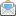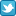# Probabilism

Probability theory may seem an attractive candidate for the foundation of rationality:

• Whereas formal logic is rarely useful in practice, probabilistic methods are indispensable in many technical fields
• Probability theory recognizes that absolute certainty is never possible in the eggplant-size world
• It provides an intuitively appealing account of greater and lesser confidence in beliefs.

Each of these addresses fatal problems in logicism. Let’s say probabilism is any rationalism that takes probabilistic rationality as central to rationality overall. “Probabilistic rationality” includes probability theory (the basic mathematics of chance), plus decision theory (a story about how you should act based on the probability of desirable outcomes), plus statistical methods.

Probabilism mostly replaced logicism as the dominant form of rationalism in the mid-twentieth century. Unfortunately:

• Probability theory lacks most of formal logic’s power
• Probabilism does not even try to address most of the issues that logicism failed at, and so also fails for the same reasons
• Probabilism’s defects are not just theoretical; they regularly produce large practical catastrophes.

From the meta-rational point of view, probabilism is wrong. However, probabilistic methods should be part of the toolkit of every rational person. Probabilistic meta-rationality means knowing when and why and how to use those methods effectively.

## Weaker and stronger probabilisms

Here are some claims about the power of probabilistic rationality, in order from weaker to stronger:

1. Probabilistic rationality is extremely valuable in some situations; use it when appropriate
2. Probabilistic rationality is a complete and correct theory of induction
3. Probabilistic rationality is a complete and correct theory of uncertainty
4. Probabilistic rationality is a complete and correct theory of epistemology
5. Probabilistic rationality is a complete and correct theory of rationality
6. Probabilistic rationality applies in all circumstances; use it always

Claim 1 is true. The rest are false. Let’s take them in reverse order.

Claims 5 and 6 can be disposed of quickly. Probabilistic rationality does not include most of mathematics; how could it be a complete theory of rationality?1 Writing a software procedure to meet a given specification is a rational activity; usually it does not involve probabilistic reasoning at all. Theoretical physics is taken as the foremost example of rationality in science; differential equations are the main tool in theoretical physics; differential equations are not part of probability theory. Probability theory can be combined with differential equations, but that doesn’t make it a complete theory of rationality, any more than calculus is a complete theory of rationality.

As an epistemology (claim 4), probabilism looks like this:2

Sentence Confidence Truth
Alain is bald 0.7384261 True
Ludwig has fed the dog 0.8729682 True
Immanuel has woken from his slumber 0.6260485 False
Self-consciousness recognizes its positive relation as its negative, and its negative as its positive; or, in other words, recognizes these opposite activities as the same, i.e. it recognizes pure Thought or Being as self-identity, and this again as separation. 0.2195923 True

This illustrates probabilism’s sharing most of the problems that defeat logicism:

• It requires that all beliefs are either absolutely true or absolutely false of the world. (Otherwise, the math doesn’t work.)
• It does not address any of the representational problems (ambiguity, vagueness, definitions, reference, propositions).
• It does not address any of the ontological problems (the nebulosity of objects, categories, properties, and relationships).
• Among logicism’s epistemological failings, probabilism addresses only uncertainty about known unknowns (not unknown unknowns, relevance, new ideas, or most issues in perception).

Probabilism simply ignores most of the issues logicism addressed. It doesn’t just have most of the same defects, it also doesn’t have most logic’s strengths. It’s a much weaker system in terms of expressive power (what it allows you to say) and inferential power (what you can conclude from a set of givens).3 The whole of mathematics is pretty much contained within logic; the same is not true for probability theory.

As a theory of uncertainty (claim 3), probabilistic rationality addresses only certain types of known unknowns. It can fail catastrophically when it encounters unknown unknowns, or known unknowns that don’t play fair. I’ll discuss that in following chapters.

Probabilism is most nearly convincing as a theory of scientific induction (claim 2). How much evidence should make you how confident about a scientific theory? Statistics can give strong guidance in many cases. However, we’ll see that statistics can’t answer the question outright, and can be powerfully misleading as well. (Also, much of science isn’t based on probability at all. Isaac Newton did some pretty decent work without it. Science rarely used statistical methods before the middle of the twentieth century.)

Probabilists may implicitly assume that induction is the only significant problem in epistemology or rationality—in which case claims 4 and 5 would be more plausible. Some reasons one could imagine induction is the main issue:

• The collapse of logical positivism was widely attributed to its failure to produce a workable theory of induction. That diagnosis ignored all the other difficulties it ran into.

• Science is often misunderstood as the process of deriving universal knowledge from data. This mistakenly neglects questions of where data comes from—the importance and complexity of experiment design, of instrument development and observational practices—and also the invention of new models and theories.

• Induction is a constant hassle for scientists in practice (“how much more data do I have to collect before I’m allowed to publish?”). Scientists can usually work around the other problems faced by rationalism by applying reasonableness. (That is the main point of Part Three.) Reasonableness, however, is famously bad at estimating degrees of confidence in beliefs.

## No alternative?

When critics point out that probabilism does not provide an adequate account of induction, probabilists often reply that there is no credible alternative. Having trounced logicism, probabilism is the last man standing.4 It has to be right, or rationality would be impossible.

The unthought assumption is that rationality requires rationalism. That is, we can’t do rationality without a proof from first principles that being rational is correct. But we do do rationality, often successfully, and we don’t have a proof.

Similarly, rationalism assumes that we couldn’t trust scientific knowledge without a first-principles proof that induction works. For that, induction would probably need to be a universally applicable procedure with a uniform justification, such as the mathematics of probability. Probability doesn’t work for that, and there is no other credible candidate; so why should we trust scientific beliefs?

Well, how do scientists derive general knowledge from specific data? Parts Three through Five of The Eggplant develop an alternative answer. In sum, if you investigate this as an empirical question instead of a philosophical one, you find that there is no uniform principle of induction. Instead, different sciences have different ways of finding different sorts of truths, all of which are reasonably (but imperfectly) reliable when used well. Some use probability; some don’t.

• 1. Some rationalists simply define “rational” as “conforming to decision theory,” in which case probabilistic rationality is a complete and correct theory of rationality by definition. But this does not line up with how “rational” is used by most scientists or engineers.
• 2. This is a “Bayesian” presentation of probabilism. Bayesianism is one of the major schools of probabilistic rationalism; “frequentism” is another, and its epistemology is rather different. I believe the same general critique applies to all probabilisms, but explaining why all versions fail would involve different details for each. That would be tedious, so mostly the discussion here uses only Bayesian language.
• 3. See my “Probability theory does not extend logic” on the Meaningness website.
• 4. Bayes or Bust, as one author put it, referring to the Bayesian version of probabilism. There are other minor rationalisms, third alternatives; but they too have fatal flaws, and fewer success stories.

To hear about new content,subscribe to my email newsletter,follow me on Twitter, use theRSS feed, or see the list of recent pages.